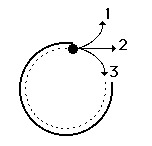Newton's Laws - Lesson 1 - Newton's First Law of Motion

# State of Motion

Inertia is the tendency of an object to resist changes in its state of motion. But what is meant by the phrase state of motion? The state of motion of an object is defined by its velocity - the speed with a direction. Thus, inertia could be redefined as follows:

Inertia: tendency of an object to resist changes in its velocity.

An object at rest has zero velocity - and (in the absence of an unbalanced force) will remain with a zero velocity. Such an object will not change its state of motion (i.e., velocity) unless acted upon by an unbalanced force. An object in motion with a velocity of 2 m/s, East will (in the absence of an unbalanced force) remain in motion with a velocity of 2 m/s, East. Such an object will not change its state of motion (i.e., velocity) unless acted upon by an unbalanced force. Objects resist changes in their velocity.

As learned in an earlier unit, an object that is not changing its velocity is said to have an acceleration of 0 m/s/s. Thus, we could provide an alternative means of defining inertia:

Inertia: tendency of an object to resist acceleration.

## Watch It!

An air track glider is shown moving across an air track. Air is blown through many small holes in the track in order to lift the glider off the track. This reduces, maybe even eliminates, the action of surface friction upon the glider. The glider moves with what seems to be a constant speed motion. As they say: objects in motion stay in motion ... .

### We Would Like to Suggest ...Sometimes it isn't enough to just read about it. You have to interact with it! And that's exactly what you do when you use one of The Physics Classroom's Interactives. We would like to suggest that you combine the reading of this page with the use of our Rocket Sledder Interactive. You can find it in the Physics Interactives section of our website. The Rocket Sledder allows a learner to explore the effect of balanced and unbalanced forces upon the acceleration of a rocket-propelled sledder.

Visit:  Rocket Sledder

### Check Your Understanding1. A group of physics teachers is taking some time off for a little putt-putt golf. The 15th hole at the Hole-In-One Putt-Putt Golf Course has a large metal rim that putters must use to guide their ball towards the hole. Mr. S guides a golf ball around the metal rim When the ball leaves the rim, which path (1, 2, or 3) will the golf ball follow?2. A 4.0-kg object is moving across a friction-free surface with a constant velocity of 2 m/s. Which one of the following horizontal forces is necessary to maintain this state of motion?

 a. 0 N b. 0.5 N c. 2.0 N d. 8.0 N e. depends on the speed.

Next Section:
Jump To Next Lesson:

Follow Us